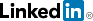cancel
Showing results for
Did you mean:Helper III

## datediff in HH:MM format

Hi,

I have two columns:
1. Start time
2. End time.

I want to know the duration of time between the two columns in hour and minute format (HH:MM)

Then I want to display their summary on the "CARD" in the same format.

Attached is a sample example.Thank you so much for helping.

BR,

Alon

1 ACCEPTED SOLUTIONAnonymous
Not applicable
Create a calculated column that for each row will store the number of seconds between the two dates. Then take the measure I've shown you and operate on the SUM of the column. So, you should create a measure like [Total Seconds] = SUM ( T[Seconds] ) and then use its value in my measure replacing the part which is responsible for calculating the seconds...

Best
Darek
12 REPLIES 12Anonymous
Not applicable
```Duration HH:MM:SS =
VAR Duration = DATEDIFF( 'Table'[StartDate], 'Table'[EndDate], SECOND )
// There are 3,600 seconds in an hour
VAR Hours =
INT ( Duration / 3600)
// There are 60 seconds in a minute
VAR Minutes =
INT ( MOD( Duration - ( Hours * 3600 ),3600 ) / 60)
// Remaining seconds are the remainder of the seconds divided by 60 after subtracting out the hours
VAR Seconds =
ROUNDUP(MOD ( MOD( Duration - ( Hours * 3600 ),3600 ), 60 ),0) // We round up here to get a whole number
// These intermediate variables ensure that we have leading zero's concatenated onto single digits
VAR H =
IF ( LEN ( Hours ) = 1,
CONCATENATE ( "0", Hours ),
CONCATENATE ( "", Hours )
)
VAR M =
IF (
LEN ( Minutes ) = 1,
CONCATENATE ( "0", Minutes ),
CONCATENATE ( "", Minutes )
)
VAR S =
IF (
LEN ( Seconds ) = 1,
CONCATENATE ( "0", Seconds ),
CONCATENATE ( "", Seconds )
)
// Now return hours, minutes and seconds with leading zeros in the proper format "hh:mm:ss"
RETURN
CONCATENATE (
H,
CONCATENATE ( ":", CONCATENATE ( M, CONCATENATE ( ":", S ) ) )
)```

This is code from:

https://community.powerbi.com/t5/Community-Blog/Aggregating-Duration-Time/ba-p/22486

Best

DarekHelper III

Hi,

The result is good, but I have to summarize the hours and minutes.
I don't seem to be able to do that because this field is text.

BR,

AlonAnonymous
Not applicable
Mate, a little bit of thinking goes a long way... If you have to summarize, then the measure should first add up all the seconds and then apply the above formula to this sum. Is this not obvious?

Best
DarekHelper III

Hi @Anonymous,

Do you have an example of such a calculation ..?Anonymous
Not applicable
Create a calculated column that for each row will store the number of seconds between the two dates. Then take the measure I've shown you and operate on the SUM of the column. So, you should create a measure like [Total Seconds] = SUM ( T[Seconds] ) and then use its value in my measure replacing the part which is responsible for calculating the seconds...

Best
DarekHelper III

Hi,

I created a calculated column that sums up the seconds between the two columns

sum_Diff_In_Second = sum(Table_Name[Diff_In_second])

Which part of your code should I replace not so clear ..?Anonymous
Not applicable

"So, you should create a measure like [Total Seconds] = SUM ( T[Seconds] ) and then use its value in my measure replacing the part which is responsible for calculating the seconds..."

Use Its value in my measure replacing the part which calculates the seconds...

I think this is as clear as the sun. Your "effort" is to find the part that calculates the seconds and substitute the value of the measure for the number of seconds. Can't be any simpler than that.

Best

DarekSuper User II

Sorry, but who are you replying too?Helper III

Hi,Super User II

The below Measure does the sum ( SUMX part ), the rest is just formatting it to DD - HH:MM:SS, technically you can use SUMX( 'Table', 'Table'[End] - 'Table'[Start] ) * 1 part on its own, however this would give you only decimal value.

```Measure =
VAR _all = SUMX( 'Table', 'Table'[End] - 'Table'[Start] ) * 1
VAR _days  = INT( _all )
VAR _time = _all - _days
RETURN  FORMAT( _days, "#0D-" ) & FORMAT( _time, "hh:mm:ss")```

Best Regards,
Mariusz

If this post helps, then please consider Accepting it as the solution.

Please feel free to connect with me.Helper III

Hi,Super User II

You can do something like below, you can adjust the code to show only hh:mm if you wish, but the below will address the issue of days when the sum goes over 24.

```Measure =
VAR _all = SUMX( 'Table', 'Table'[End] - 'Table'[Start] ) * 1
VAR _days  = INT( _all )
VAR _time = _all - _days
RETURN  FORMAT( _days, "#0D-" ) & FORMAT( _time, "hh:mm:ss")```

Best Regards,
Mariusz

If this post helps, then please consider Accepting it as the solution.

Please feel free to connect with me.